JEE  >  NCERT Solutions Exercise 5.5: Continuity & Differentiability

# NCERT Solutions Exercise 5.5: Continuity & Differentiability | Mathematics For JEE

 1 Crore+ students have signed up on EduRev. Have you?

Continuity & Differentiability

Question 1: Differentiate the function with respect to x.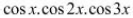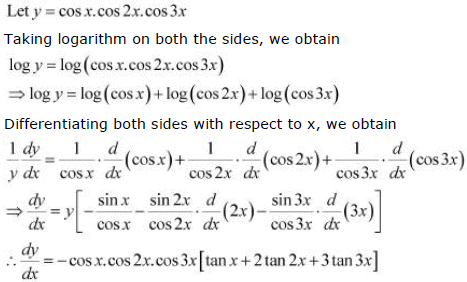Question 2: Differentiate the function with respect to x.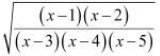Let y =Taking logarithm on both the sides, we obtain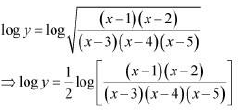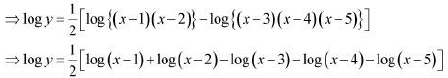Differentiating both sides with respect to x, we obtain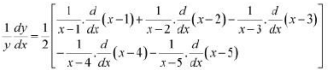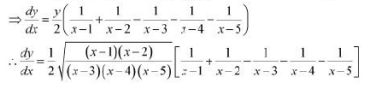Question 3: Differentiate the function with respect to x.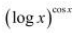Let y =Taking logarithm on both the sides, we obtain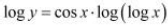Differentiating both sides with respect to x, we obtain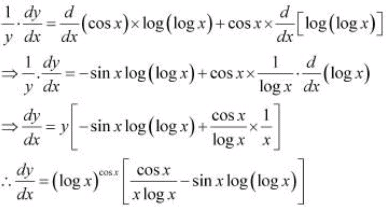Question 4: Differentiate the function with respect to x. xx - 2sinx

Let y =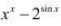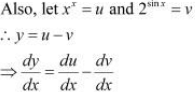u = xx
Taking logarithm on both the sides, we obtain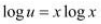Differentiating both sides with respect to x, we obtain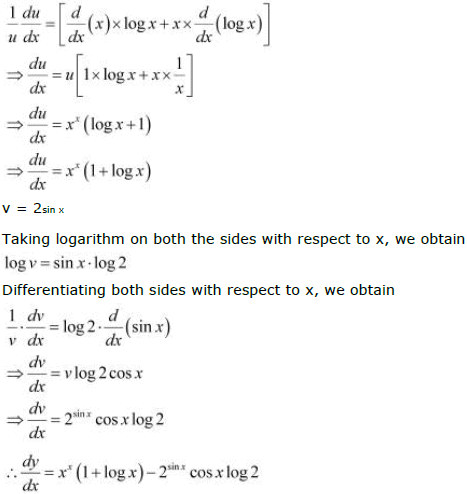Question 5: Differentiate the function with respect to x.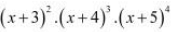Let y =Taking logarithm on both the sides, we obtain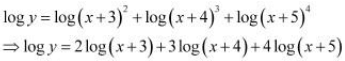Differentiating both sides with respect to x, we obtain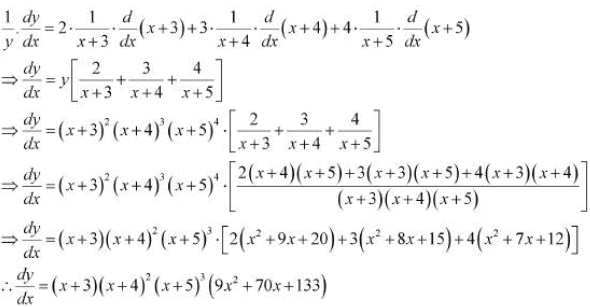Question 6: Differentiate the function with respect to x.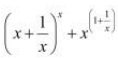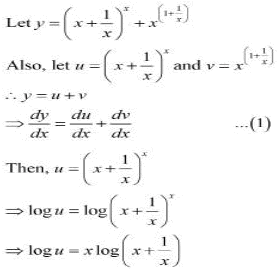Differentiating both sides with respect to x, we obtain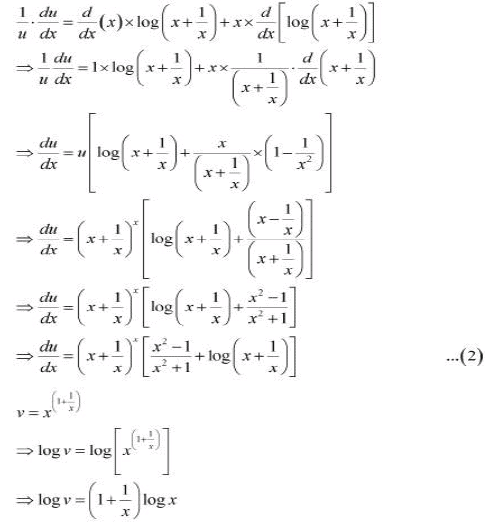Differentiating both sides with respect to x, we obtain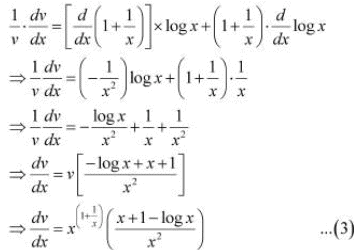Therefore, from (1), (2), and (3), we obtain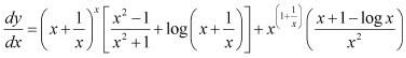Question 7: Differentiate the function with respect to x.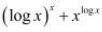Let y =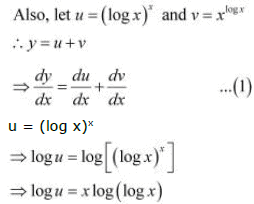Differentiating both sides with respect to x, we obtain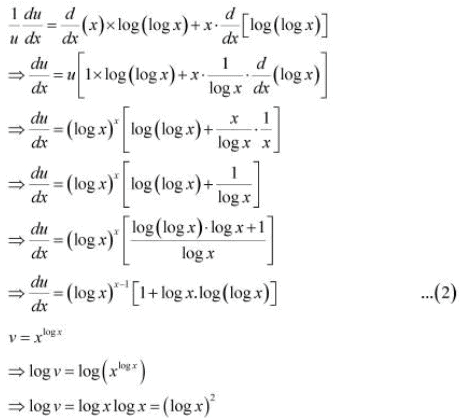Differentiating both sides with respect to x, we obtain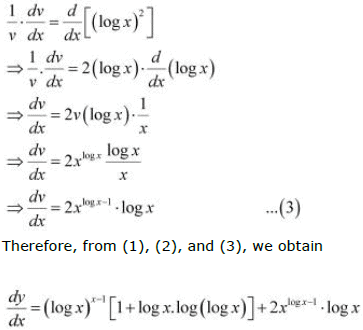Question 8: Differentiate the function with respect to x.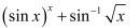Let y =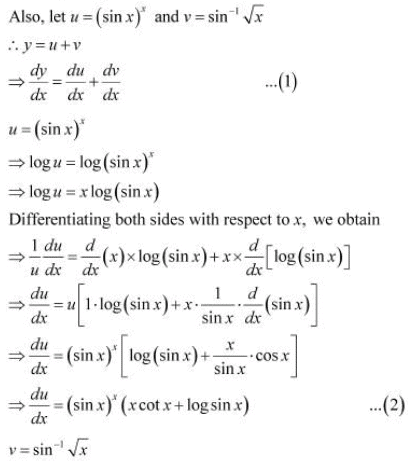Differentiating both sides with respect to x, we obtain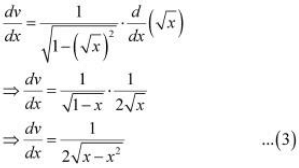Therefore, from (1), (2), and (3), we obtain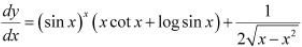Question 9: Differentiate the function with respect to x.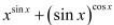Let y =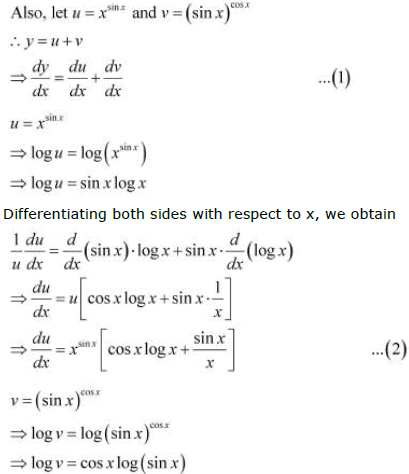Differentiating both sides with respect to x, we obtain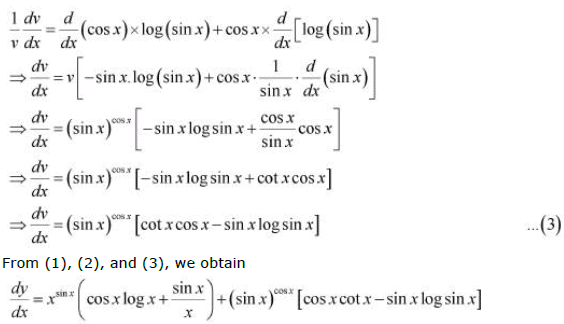Question 10: Differentiate the function with respect to x.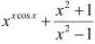Let y =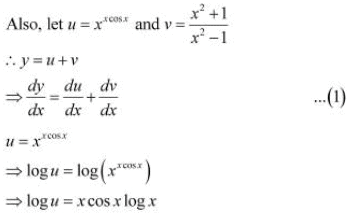Differentiating both sides with respect to x, we obtain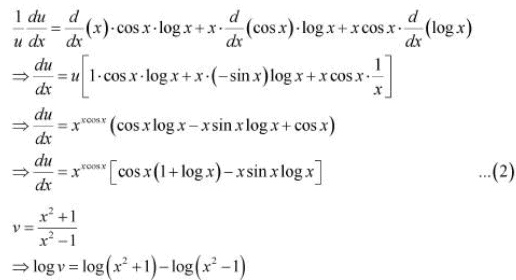Differentiating both sides with respect to x, we obtain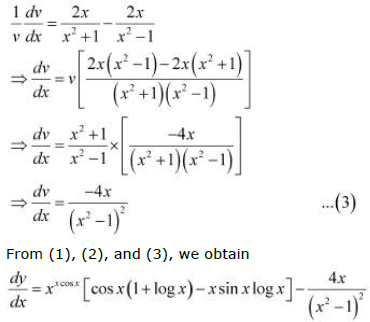Question 11: Differentiate the function with respect to x.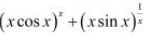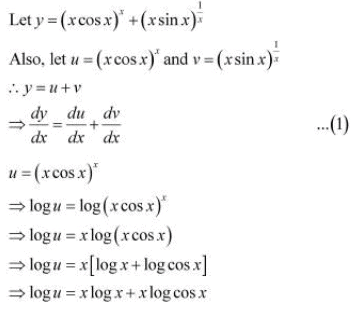Differentiating both sides with respect to x, we obtain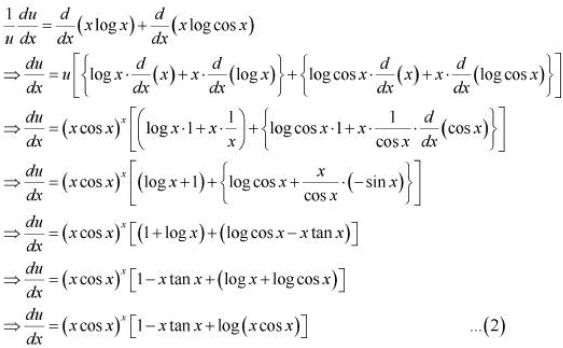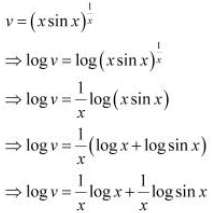Differentiating both sides with respect to x, we obtain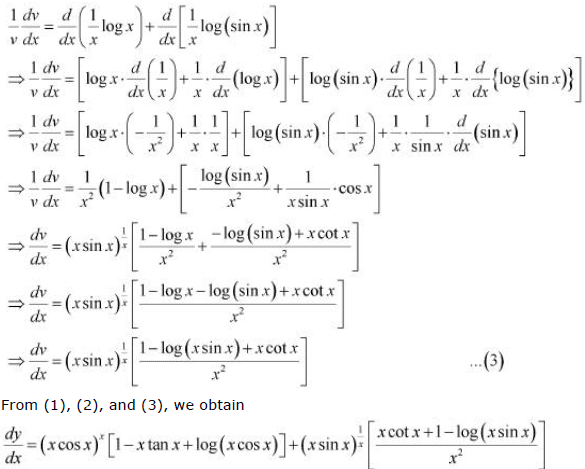Question 12:
Find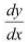of function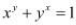.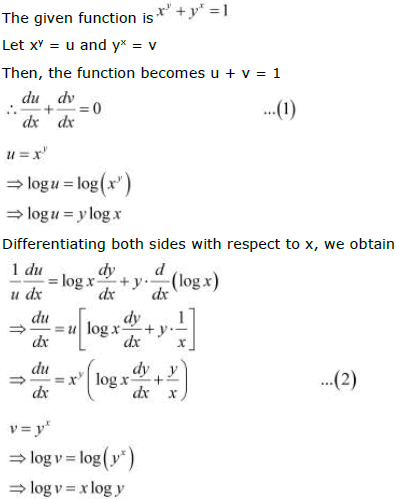Differentiating both sides with respect to x, we obtain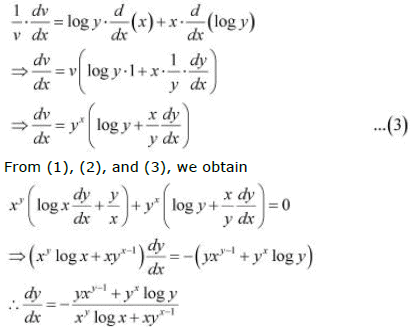Question 13:

Find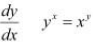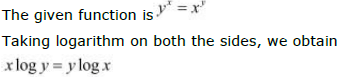Differentiating both sides with respect to x, we obtain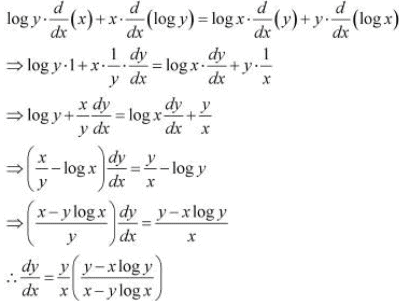Question 14:
Findof function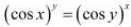.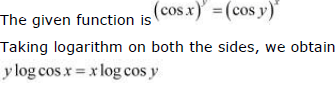Differentiating both sides, we obtain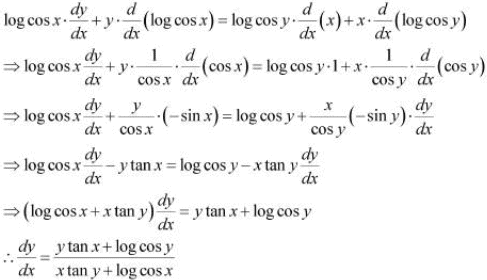Question 15:
Findof function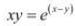.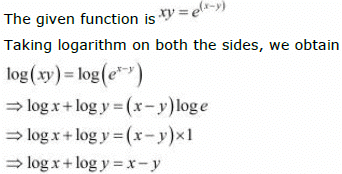Differentiating both sides with respect to x, we obtain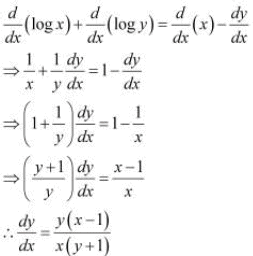Question 16:
Find the derivative of the function given by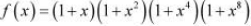and
hence find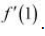.

The given relationship isTaking logarithm on both the sides, we obtain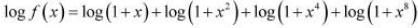Differentiating both sides with respect to x, we obtain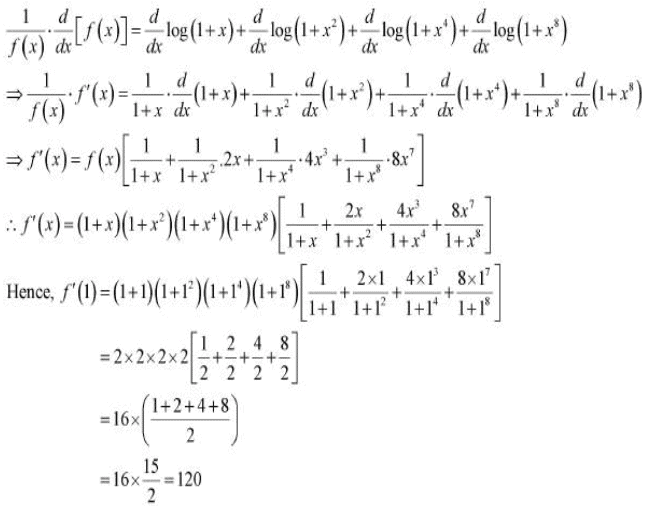Question 17:
Differentiate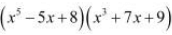in three ways mentioned below
(i) By using product rule.
(ii) By expanding the product to obtain a single polynomial.
(iii By logarithmic differentiation.
Do they all give the same answer?

Let y =(i)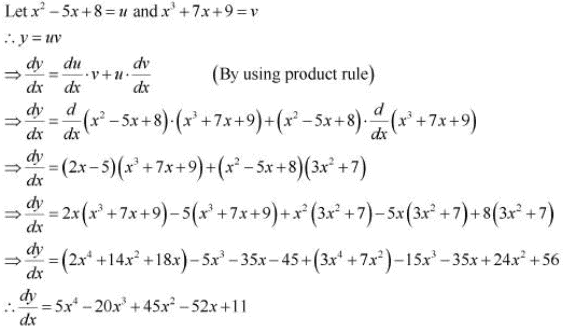(ii)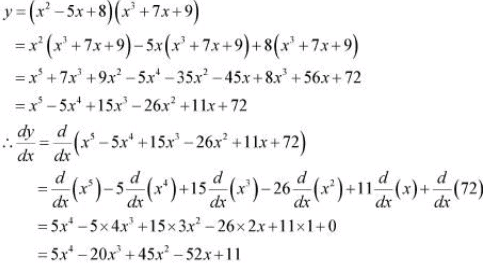( iii)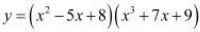Taking logarithm on both the sides, we obtain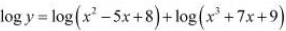Differentiating both sides with respect to x, we obtain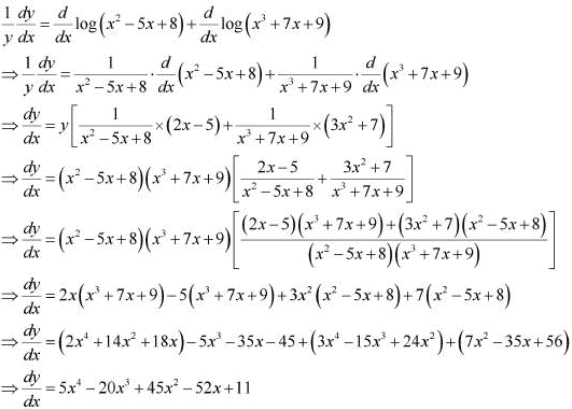From the above three observations, it can be concluded that all the results ofare same.

Question 18: If u, v and w are functions of x, then show that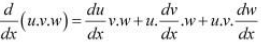in two ways-first by repeated application of product rule, second by logarithmic
differentiation.

Let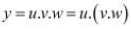By applying product rule, we obtain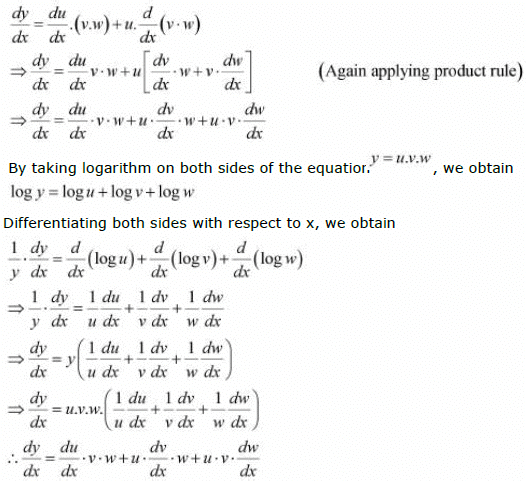The document NCERT Solutions Exercise 5.5: Continuity & Differentiability | Mathematics For JEE is a part of the JEE Course Mathematics For JEE.
All you need of JEE at this link: JEE

## Mathematics For JEE

130 videos|359 docs|306 tests

## Mathematics For JEE

130 videos|359 docs|306 tests

Track your progress, build streaks, highlight & save important lessons and more!(Scan QR code)

,

,

,

,

,

,

,

,

,

,

,

,

,

,

,

,

,

,

,

,

,

;